# 机器学习-吴恩达：学习笔记及总结（4）

## Coursera | Machine Learning | Andrew Ng

Thistledown    March 10, 2020

Course Link ：Week 4 - Neural Networks: Representation

## 11 Motivations

#### 11.1 Non-linear Hypotheses

Neural Networks is a much better way to learn complex non-linear hypotheses even when your input feature space $(n)$ is large.

#### 11.2 Neurons and the Brain

• Origins: Algorithms that try to mimin the brain.
• Was very widely used in 80s and early 90s; popularity diminished in late 90s.
• Recent resurgence: State-of-the-art technique for many applications.

## 12 Neural Networks

#### 12.1 Model Representation I

• Neuron in the brain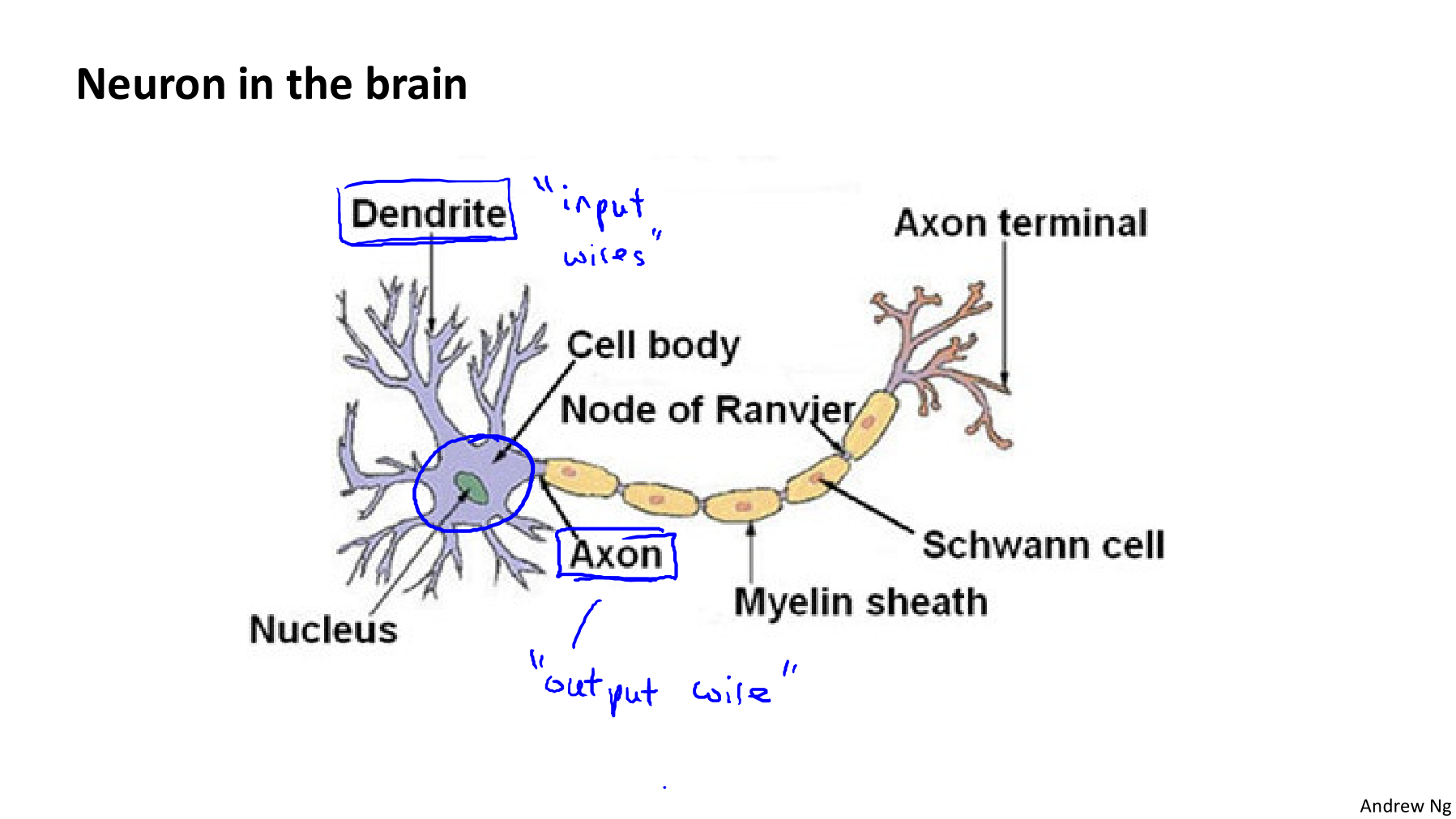• Neurons are basically computational units that take inpus (dendrites) as electrical inputs (called “spikes”) that are channeled to outputs (axons).
• Neuron model: Logistic unit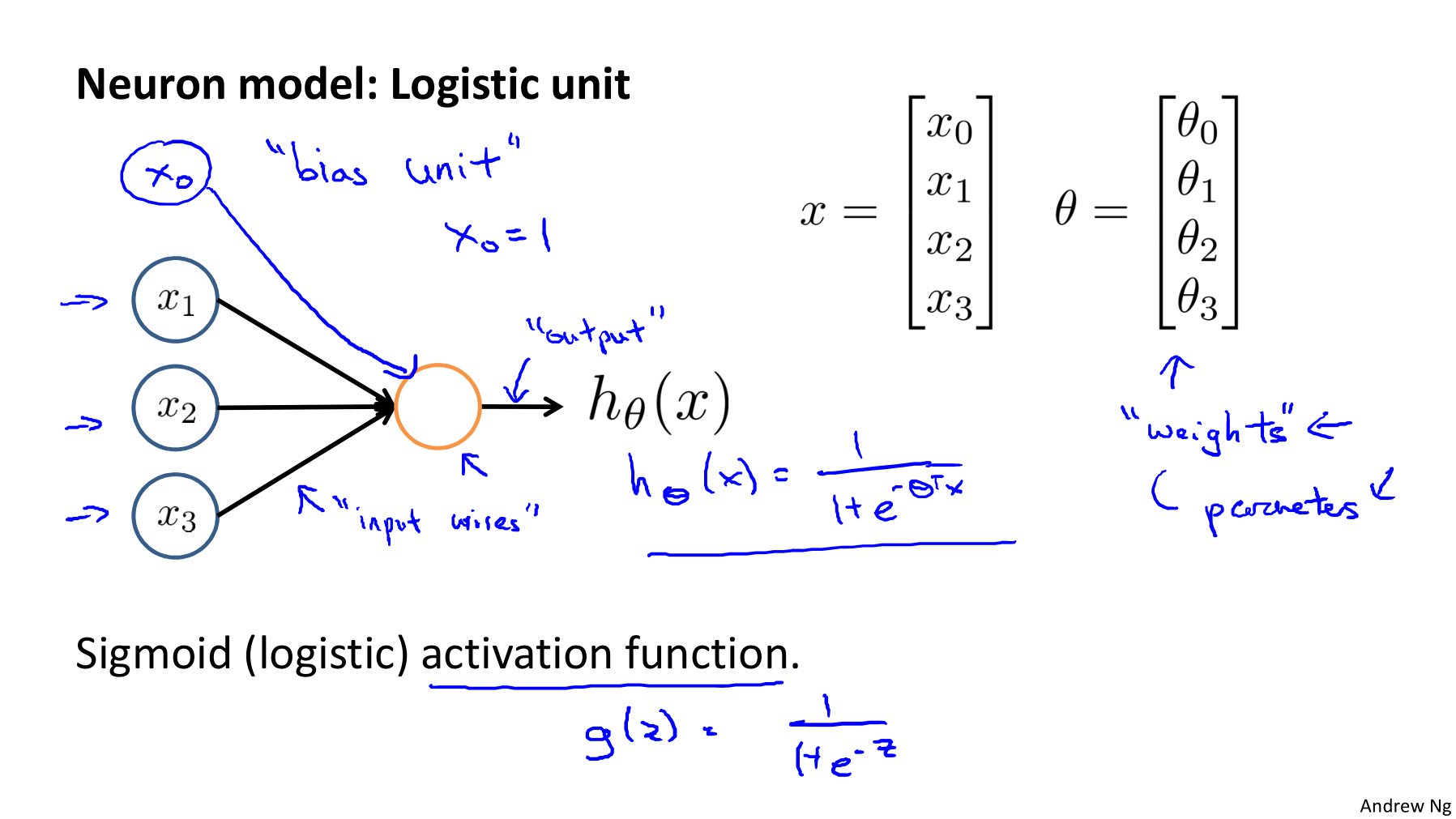• In our model, our dendrites are like the input features $x_1 \cdots x_n$, and the output is the result of our hypothesis function.

• In this model our $x_0$ input node is sometimes called the “bias unit”, it is always equal to 1.

• In neural networks, we use the same logistic function as in classification, $\frac{1}{1+e^{-\theta^Tx}}$, yet we sometimes call it a sigmoid (logistic) activation function.

• In this situation, our “$\theta$” parametrs are sometimes called “weights”.

• Visually, a simplistic representation looks like:

• Neural Network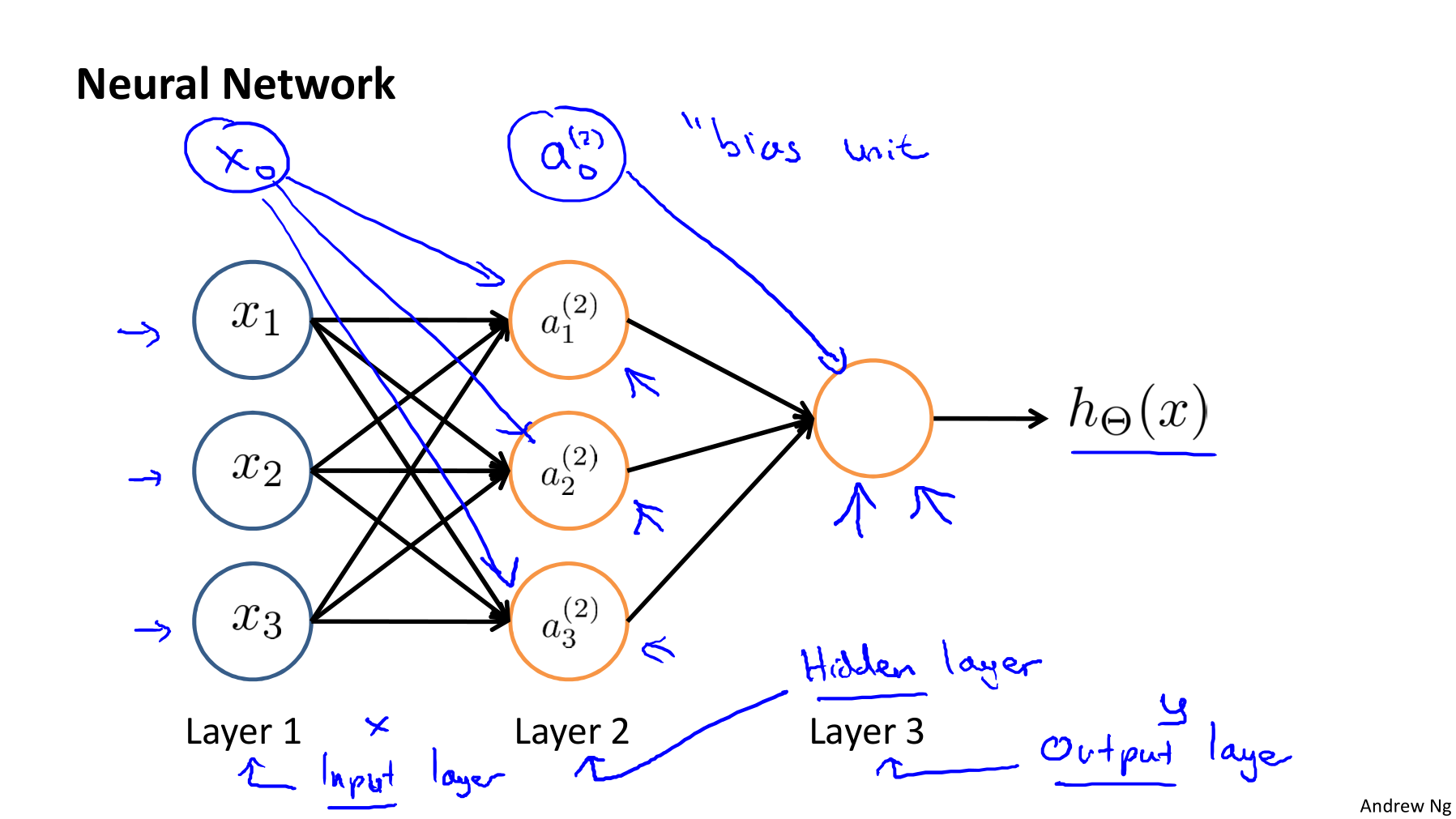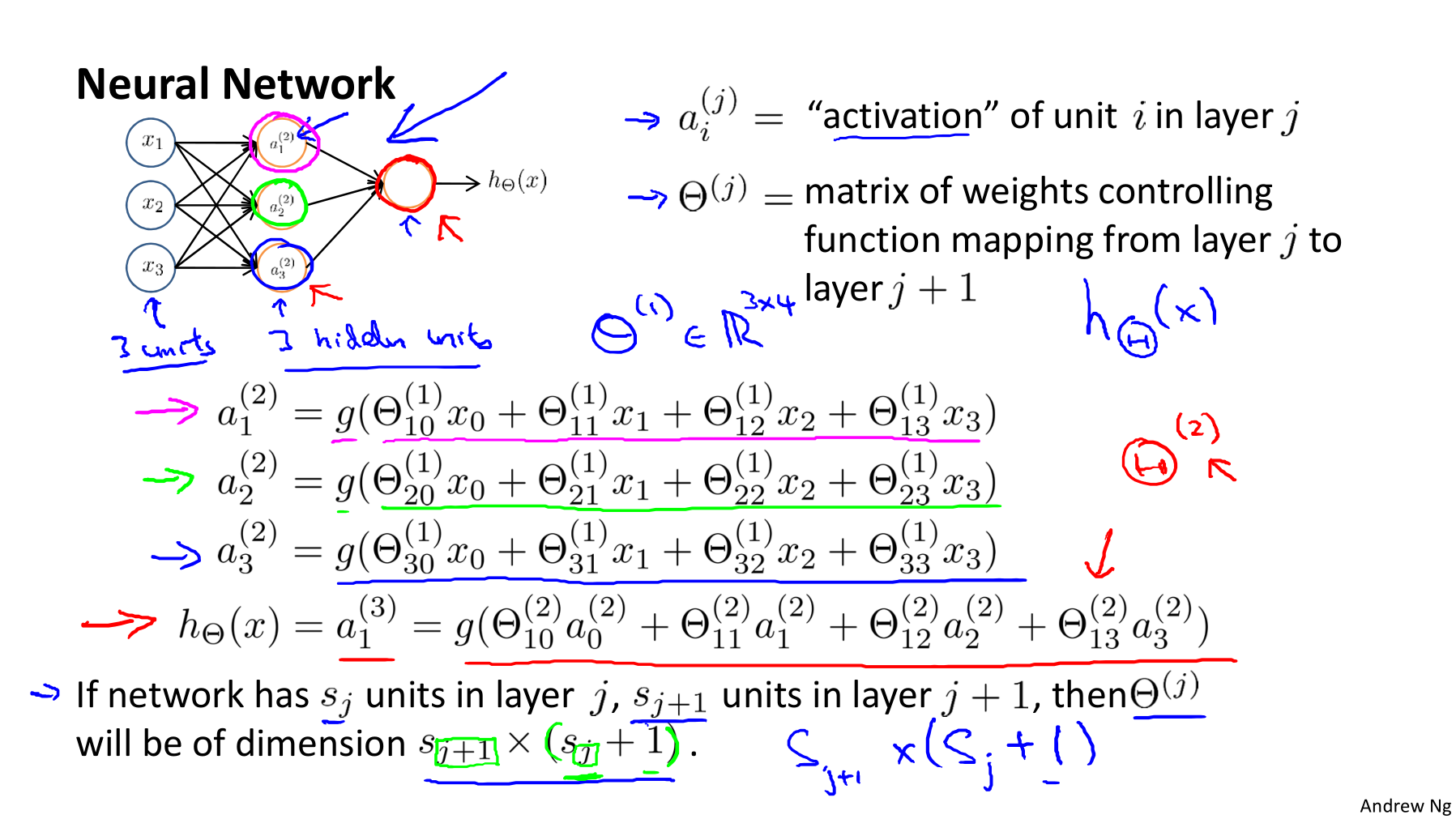• Our input nodes (layer 1), also known as the “input layer”, go into another node (layer 2), which finally outputs the hypothesis function, known as the “output layer”.

• We can have intermediate layers of nodes between the input and output layers called the “hidden layers”.

• In this example, we label these intermediate or “hidden” layer nodes $a_0^2 \cdots a_n^2$ and call them “activation units”.

• If we had one hidden layer, it would look like:

• The values for each of the “activation” nodes is obtained as follows:

• This is saying that we compute our activation nodes by using a $3\times4$ matrix of parameters. We apply each row of the parameters to our inputs to obtain the value for one activation node. Our hypothesis output is the logistic function applied to the sum of the values of our activation nodes, which have been multiplied by yet another parameter matrix $\Theta^{(2)}$ containing the weights for our second layer of nodes.

• Each layer gets its own matrix of weights, $\Theta^{(j)}$.

• The dimensions of these matrices of weights is determined as follows:

The $+1$ comes from the addition in $\Theta^{(j)}$ of the “bias nodes”, $x_0$ and $\Theta_0^{(j)}$. In other words, the output nodes will not include the bias nodes while the inputs will.

#### 12.2 Model Representation II

• Forward propation: Vectorized implementation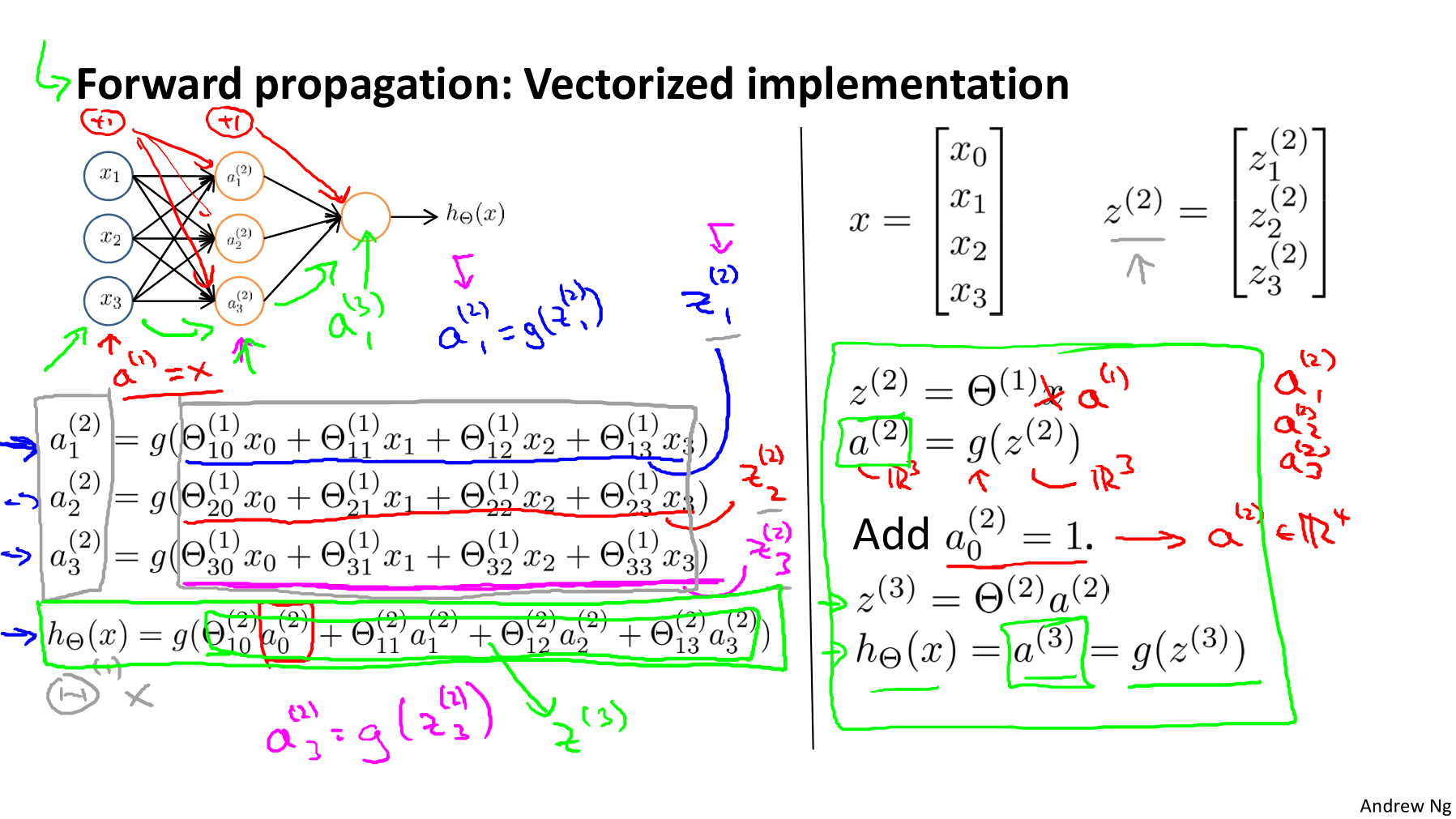• To re-iterate, the following is an example of a neural network:

• In this section we’ll do a vectorized implementation of the above functions. We’re going to define a new variable $z_k^{(j)}$ that encompasses the parameters inside our $g$ function.

• In our previous example, if we replaced by the variable $z$ for all the parameters we would get:

• In other words, for layer $j=2$ and node $k$, the variable $z$ will be:

• The vector represation of $x$ and $z^{(j)}$ is:

• Setting $x=a^{(1)}$, we can rewrite the equation as: $z^{(j)}=\Theta^{(j-1)}a^{(j-1)}$

• We are multiplying our matrix $\Theta^{(j-1)}$ with dimensions $s_j \times (n+1)$ (where $s_j$ is the number of our activation nodes) by our vector $a^{(j-1)}$ with height $(n+1)$. This gives us our vector $z^{(j)}$ with height $s_j$.

• Now we can get a vector of our activation nodes for layer $j$ as follows: $a^{(j)} = g(z^{(j)})$. Where our function $g$ can be applied element-wise to our vector $z^{(j)}$.

• We can then add a bias unit (equal to 1) to layer $j$ after we have computed $a^{(j)}$. This will be element $a_0^{(j)}$ and will be equal to 1.

• To compute our final hypothesis, let’s first compute another $z$ vector:

• We get this final $z$ vector by multiplying the next theta matrix after $\Theta^{(j-1)}$ with the values of all the activation nodes we just got.

• This last theta matrix $\Theta^{(j)}$ will have only one row which is multiplied by one column $a^{(j)}$ so that our result is a single number. We then get our final result with:

• Notice that in this last step, between layer $j$ and layer $j+1$, we are doing exactly the same thing as we did in logistic regression. Adding all these intermediate layers in neural networks allows us to more elegantly produce interesting and more complex non-linear hypothesis.
• Neural Network learning its own features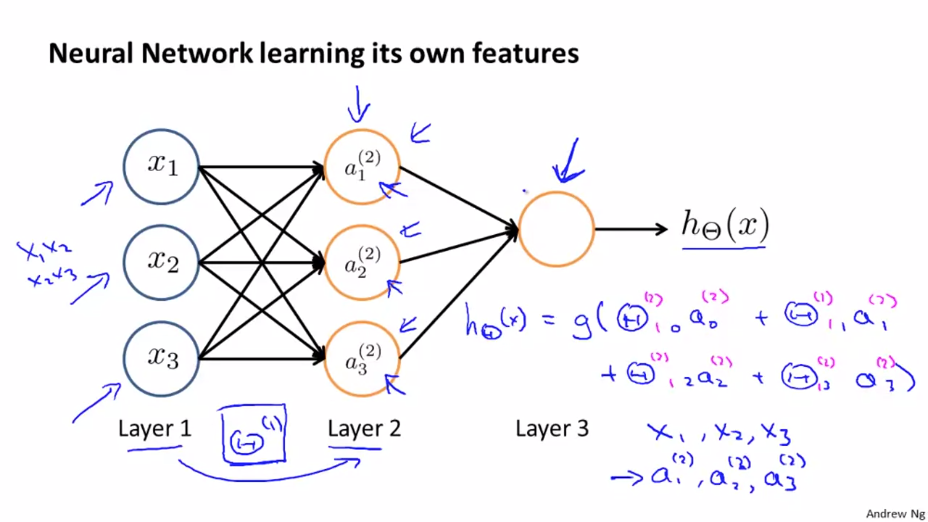• Other network architectures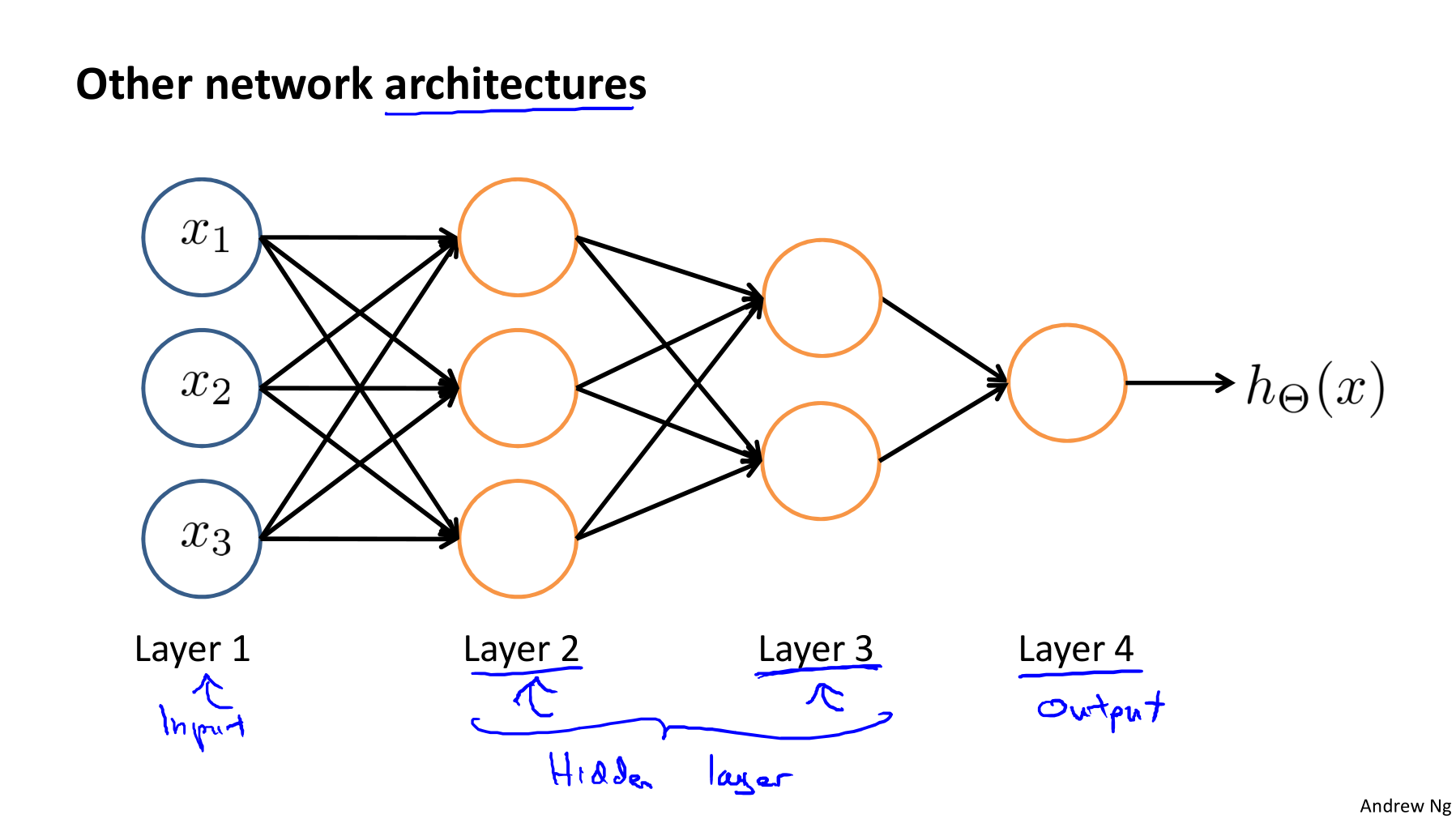## 13 Applications

#### 13.1 Examples and Intuitions I

• Non-linear classification examples: XOR/XNOR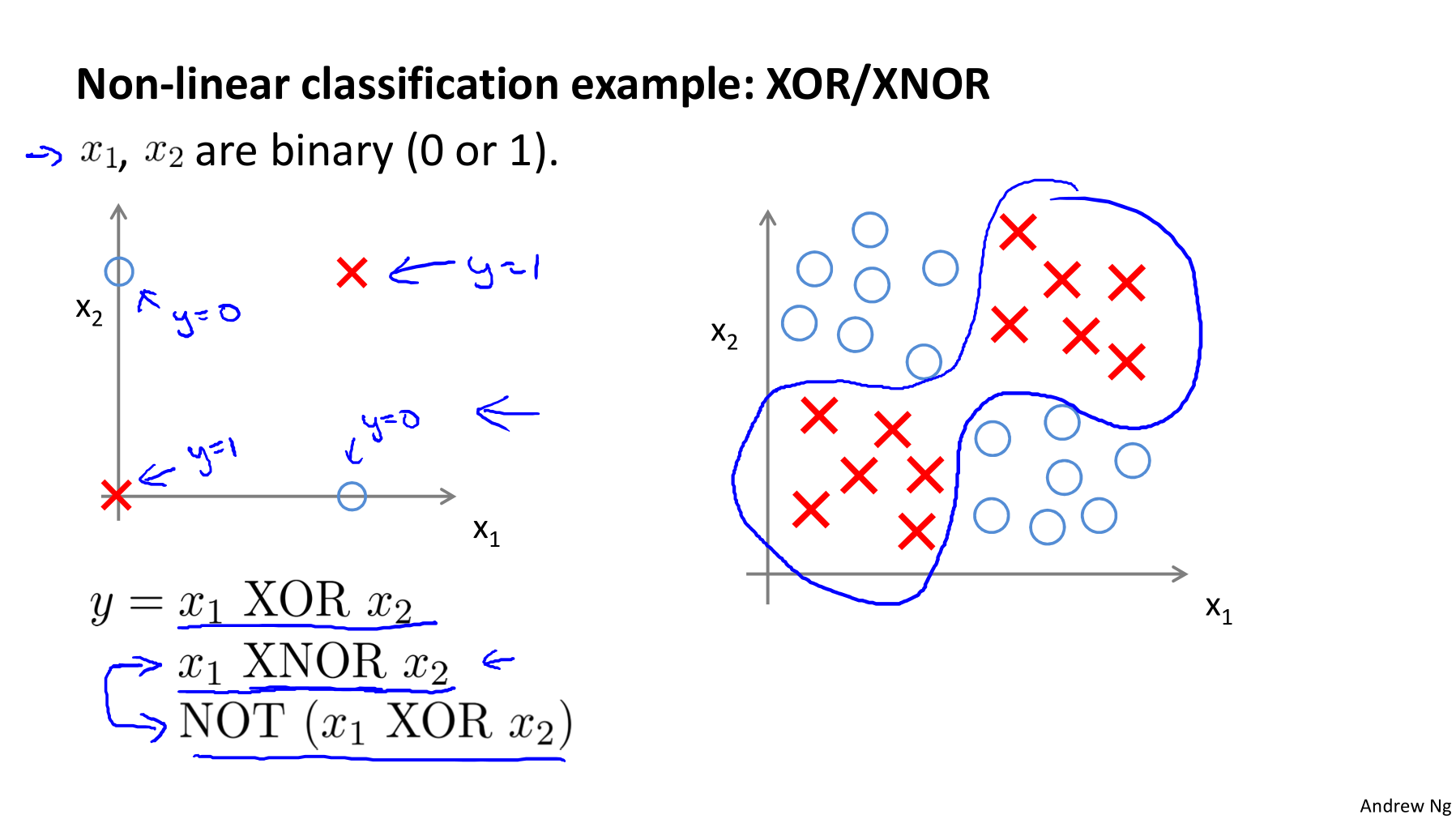• Simple example: AND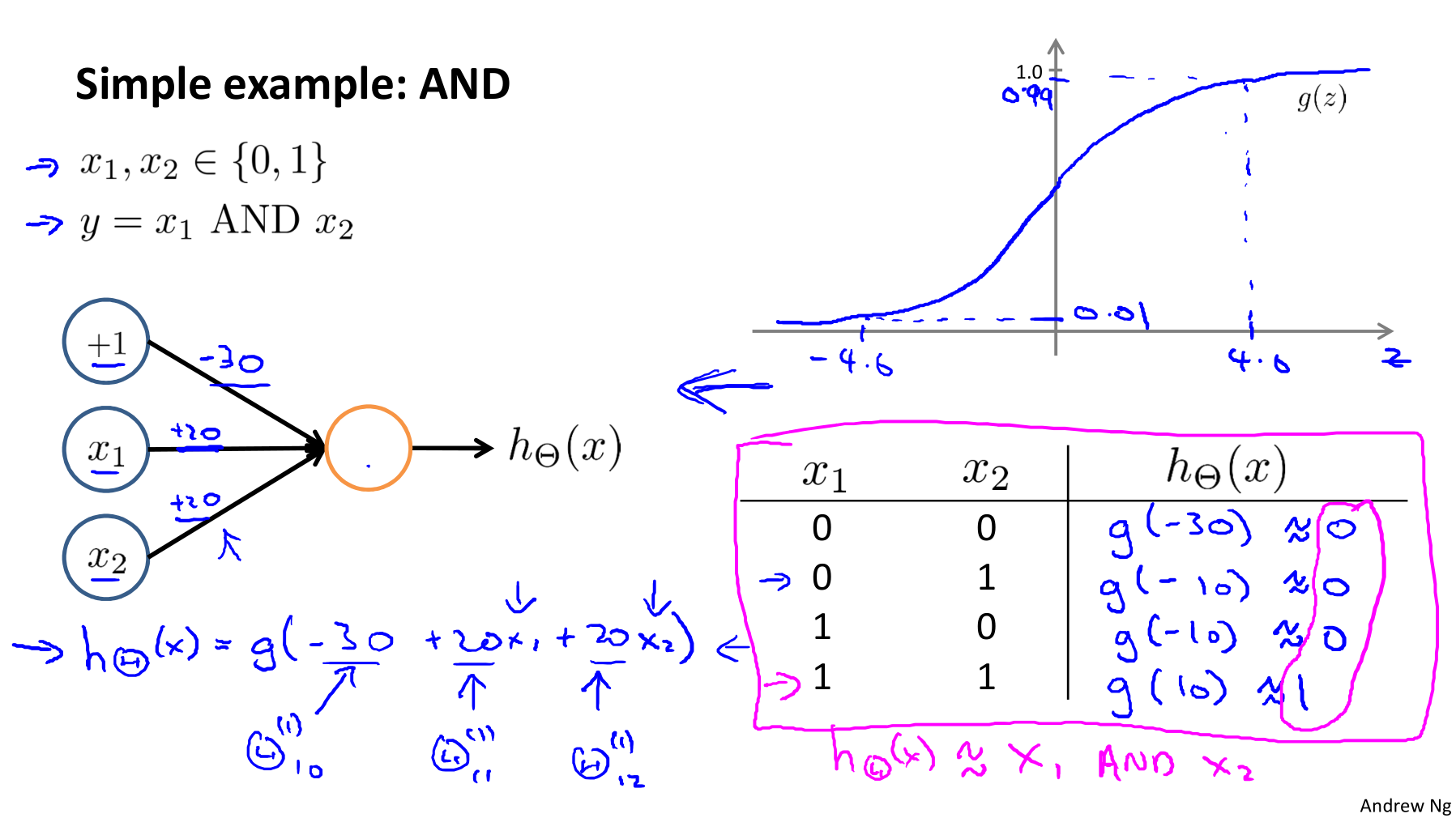• A simple example of applying neural networks is predicting $x_1 \text{AND } x_2$ , which is the logical ‘and’ operator and is only true if both $x_1$ and $x_2$ are 1.

• The graph of our function will look like:

Remember that $x_0$ is our bias variable and is always 1.

• Let’s set our first theta matrix as: $\Theta^{(1)} = \begin{bmatrix} -30 & 20 & 20 \end{bmatrix}$.

• This will cause the output of our hypothesis to only be positive if both $x_1$ and $x_2$ are 1. In other words:

• So we have constructed one of the fundamental operations in computers by using a small neural network rather than using an actual AND gate.

• Neural networks can also be used to simulate all the other logical gates. The following is an example of the logical operator ‘OR’, meaning either $x_1$ is true or $x_2$ is ture, or both.Where $g(z)$ is the following: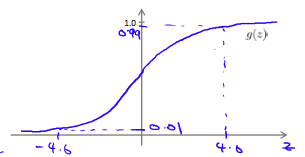#### 13.2 Examples and Intuitions II

• Negation ($\text{NOT } x_1$):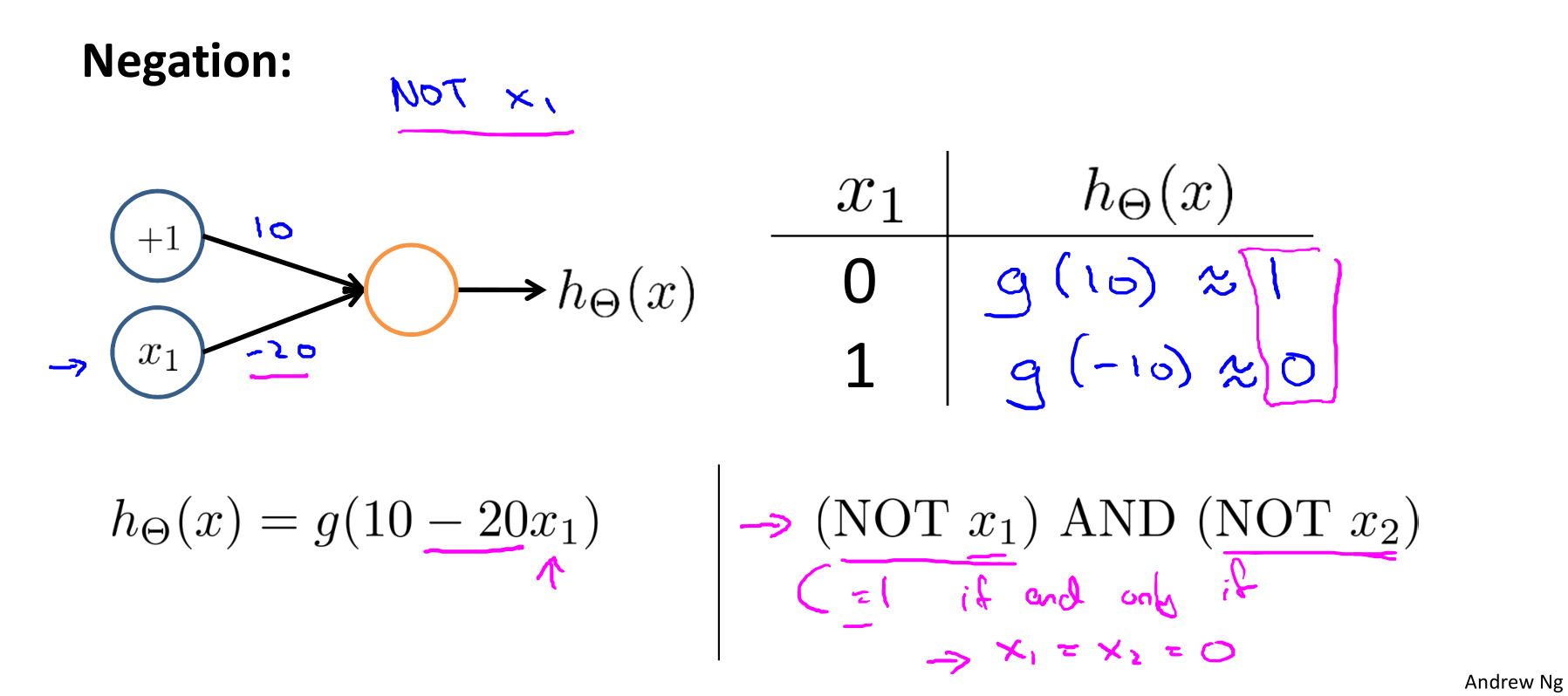• Putting it together: $x_1 \text{ XNOR } x_2$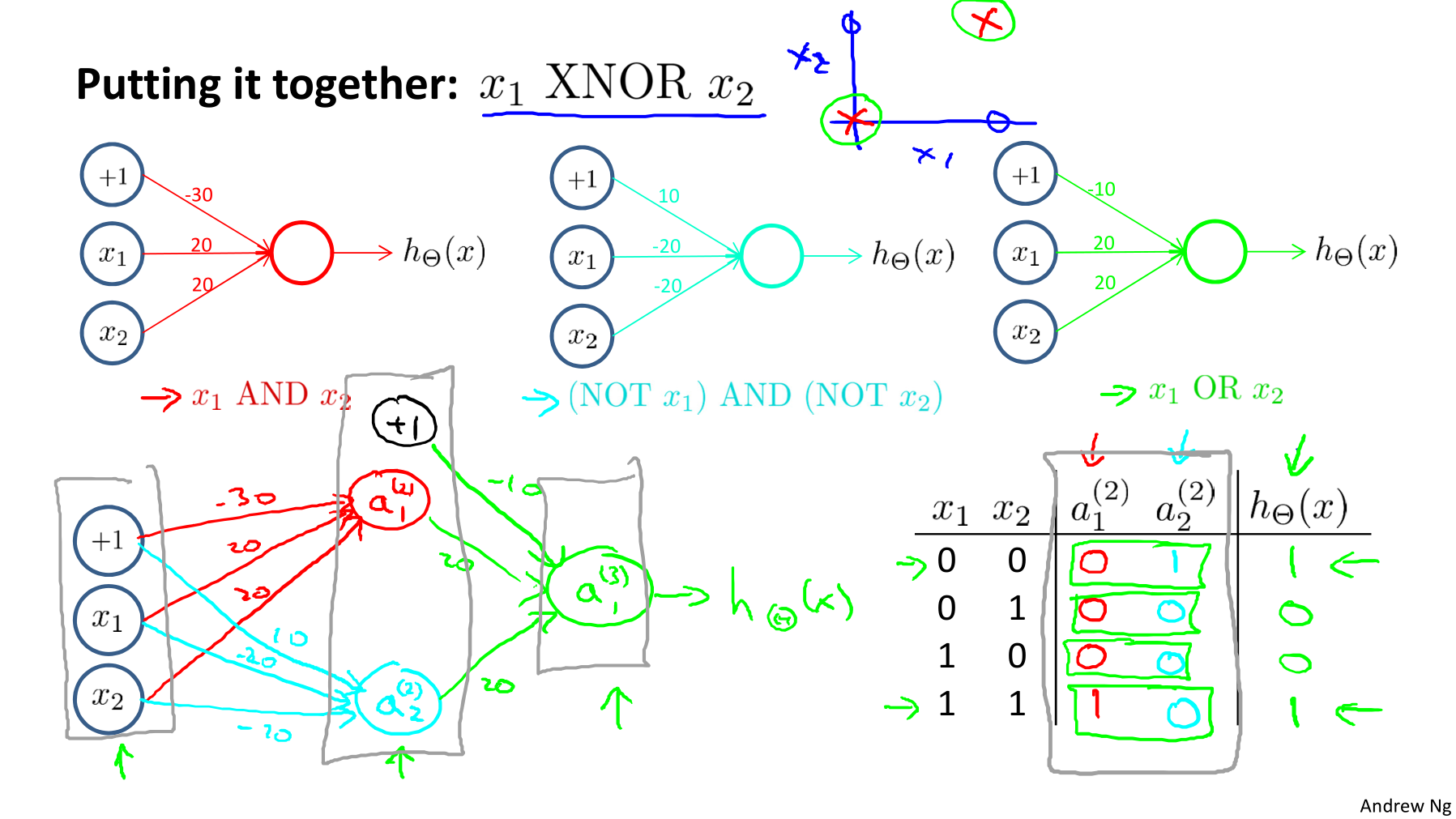• The $\Theta^{(1)}$ matrices for AND, NOR, and OR are:

• We can combine these to get the XNOR logical operator (which gives 1 if $x_1$ and $x_2$ are both 0 or both 1).

• For the transition between the first and second layer, we’ll use a $\Theta^{(1)}$ matrix that combines the values for AND and NOR:

• For the transition between the second and third layer, we’ll use a $\Theta^{(2)}$ matrix that uses the value for OR:

• Let’s write out the values for all our nodes:

• And there we have the XNOR operator using a hidden layer with two nodes.

• Neural Network intuition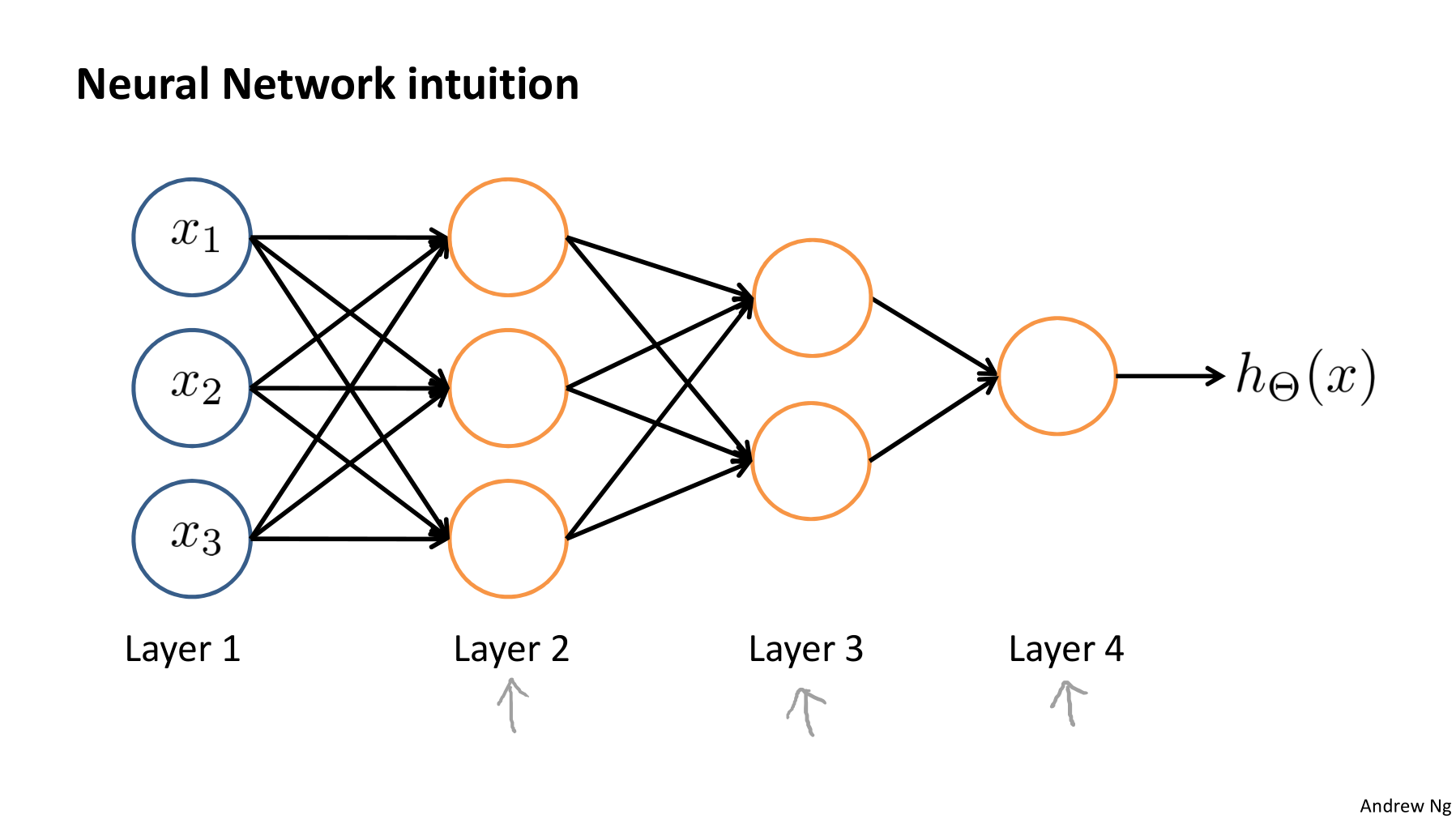• Examples: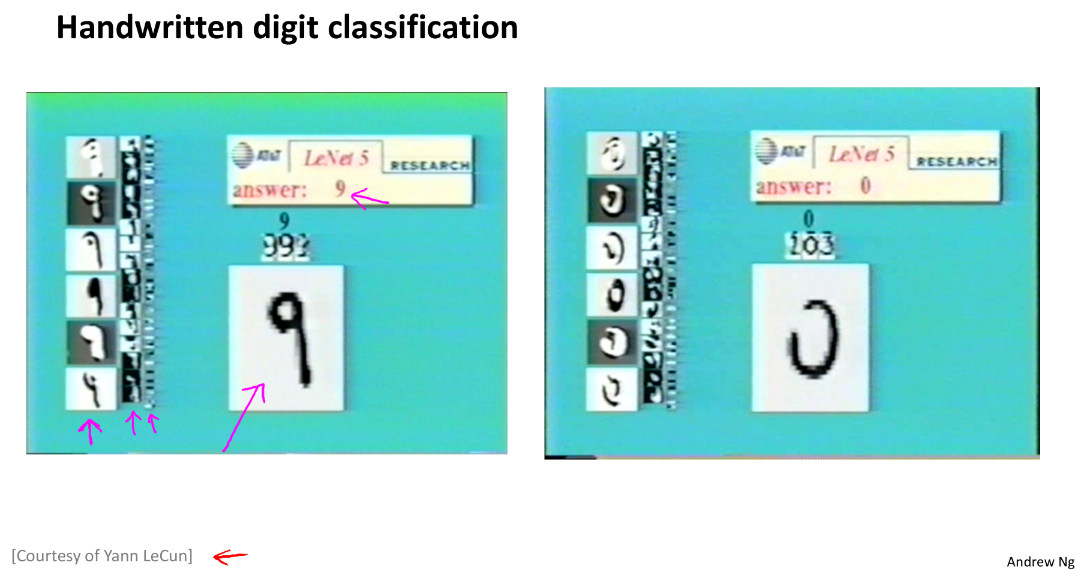#### 13.3 Multiclass Classification

• Multiple output units: One-vs-all

• To classify data into multiple classes, we let our hypothesis function return a vector of values. Say we wanted to classify our data into one of four categories. We will use the following example to see how this classification is done. This algorithm takes as input an image and classifies it accordingly.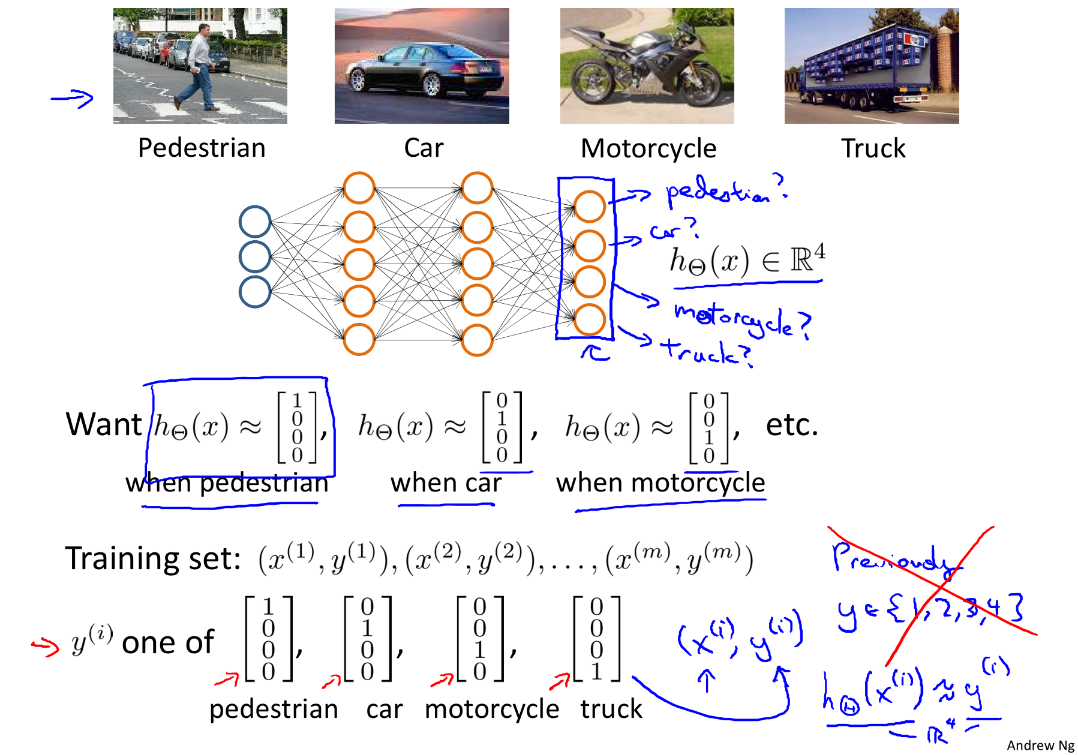• We can define our set of resulting classes as $y$:

• Each $y^{(i)}$ represents a different image corresponding to either a car, pedestrian, truck, or motorcycle. The inner layers, each provides us with some new information which leads to our final hypothesis function.

• The setup looks like:

• Our resulting hypothesis for one set of inputs may look like:

In which case our resulting class is the third one down, or $h_\Theta(x)_3$, which represents the motorcycle.

## Ex3: Multi-class Classification and Neural Networks👨‍💻

See this exercise on Coursera-MachineLearning-Python/ex3/ex3.ipynb

#### Ex3.1 Multi-class Classification

Instruction: For this exercise, you will use logistic regression and neural networks to recognize handwritten digits (from 0 to 9). Automated handwritten digit recognition is widely used today - from recognizing zip codes (postal codes) on mail envelopes to recognizing amounts written on bank checks. This exercise will show you how the methods you’ve learned can be used for this classification task.

Code:

1
2
3
4
5
6
7
8
9
10
11
12
13
14
15
16
17
18
19
20
21
22
23
24
25
26
27
28
29
30
31
32
33
34
35
36
37
38
39
40
41
42
43
44
45
46
47
48
49
50
51
52
53
54
55
56
57
58
59
60
61
62
63
64
65
66
67
68
69
70
71
72
73
74
75
76
77
78
79
80
81
82
83
84
85
86
87
88
89
90
91
92
93
94
95
96
97
98
99
100
101
102
103
104
105
106
107
108
109
110
111
112
113
114
import numpy as np
import matplotlib.pyplot as plt
import scipy.io as scio
import scipy.optimize as opt

''' Part 0: Functions and Parameters '''
X, y = data['X'], data['y']
m = len(y)
return X, y, m

# Randomly select several data points to display
def randomDisplay(data, num=100, cmap='binary', transpose=True):
sample = data[np.random.choice(len(data), num)]
size, size1 = int(np.sqrt(num)), int(np.sqrt(sample.shape))
fig0, ax0 = plt.subplots(nrows=size, ncols=size, sharex=True, sharey=True, figsize=(8, 8))
order = 'F' if transpose else 'C'
for i in range(size):
for j in range(size):
ax0[i, j].imshow(sample[size * i + j].reshape([size1, size1], order=order), cmap=cmap)
plt.xticks(np.array([]))
plt.yticks(np.array([]))
plt.suptitle(str(num)+' examples from the dataset', fontsize=24)

# Sigmoid function
def sigmoid(z):
return 1 / (1 + np.exp(-z))

# Compute cost and gradient for logistic regression with regularization
def lrCostFunction(theta, X, y, lam):
m = len(y)
cost1 = - np.sum(y * np.log(sigmoid(np.dot(X, theta))) + (1 - y) * np.log(1 - sigmoid(np.dot(X, theta)))) / m
cost2 = 0.5 * lam * np.dot(theta[1:].T, theta[1:]) / m
cost = cost1 + cost2
grad = np.dot(X.T, sigmoid(np.dot(X, theta)) - y) / m
grad[1:] += (lam * theta / m)[1:]

def costFunc(param, *args):
X, y, lam = args
m, n = X.shape
theta = param.reshape([n, 1])
cost1 = - np.sum(y * np.log(sigmoid(np.dot(X, theta))) + (1 - y) * np.log(1 - sigmoid(np.dot(X, theta)))) / m
cost2 = 0.5 * lam * np.dot(theta[1:].T, theta[1:]) / m
return cost1 + cost2

X, y, lam = args
m, n = X.shape
theta = param.reshape(-1, 1)
grad = np.dot(X.T, sigmoid(np.dot(X, theta)) - y) / m
grad[1:] += (lam * theta / m)[1:]

# oneVsAll() trains multiple logistic regression classifiers and returns all
# the classifiers in a matrix all_theta, where the i-th row of all_theta
# corresponds to the classifier for label i
def oneVsAll(X, y, num_labels, lam):
m, n = X.shape
all_theta = np.zeros([num_labels, n + 1])
X = np.c_[np.ones([m, 1]), X]
for i in range(1, num_labels + 1):
params = np.zeros(n + 1)
args = (X, y == i, lam)
res = opt.minimize(fun=costFunc, x0=params, args=args, method='TNC', jac=gradFunc)
all_theta[i - 1, :] = res.x

return all_theta

# Predict the label for a trained one-vs-all classifier
def predictOneVsAll(X, all_theta):
m, n = X.shape
X = np.c_[np.ones([m, 1]), X]
h_argmax = np.argmax(sigmoid(np.dot(X, all_theta.T)), axis=1)
return h_argmax + 1

# Setup the parameters
input_layer_size = 400  # 20x20 Input Images of Digits
num_labels = 10         # 10 labels, form 1 to 10 (note that we have mapped '0' to label '10')

# Randomly select 100 data points to display
randomDisplay(X, 100)
plt.show()

''' Part 2.1: Vectorize Logistic Regression '''
# Test case for lrCostFunction
print('\nTesting lrCostFunction() with regularization')
theta_test = np.array(([-2], [-1], , ))
X_test = np.c_[np.ones([5, 1]), np.linspace(1, 15, 15).reshape([5, 3], order='F') / 10]
y_test = np.array((, , , , ))
lam_test = 3

cost, grad = lrCostFunction(theta_test, X_test, y_test, lam_test)

print('Cost: ', cost.flatten())
print('Expected cost: 2.534819')
print('Expected gradients: 0.146561\t -0.548558\t 0.724722\t 1.398003')

''' Part 2.2: One-vs-All Training '''
print('\nTraining One-vs-All Logistic Regression...')
theta = oneVsAll(X, y, num_labels=num_labels, lam=1.0)

''' Part 3: Predict for One-Vs-All '''
y_predict = predictOneVsAll(X, all_theta=theta)
print('Training Set Accuracy: ', np.mean(y.ravel() == y_predict) * 100, '%')


Output:

• Console: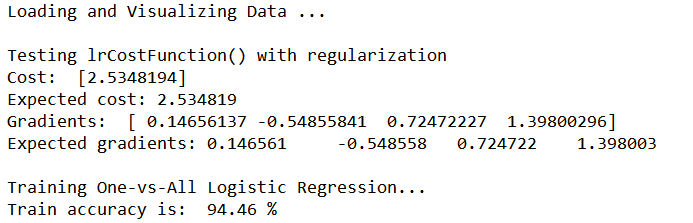• Randomly select several data points to display: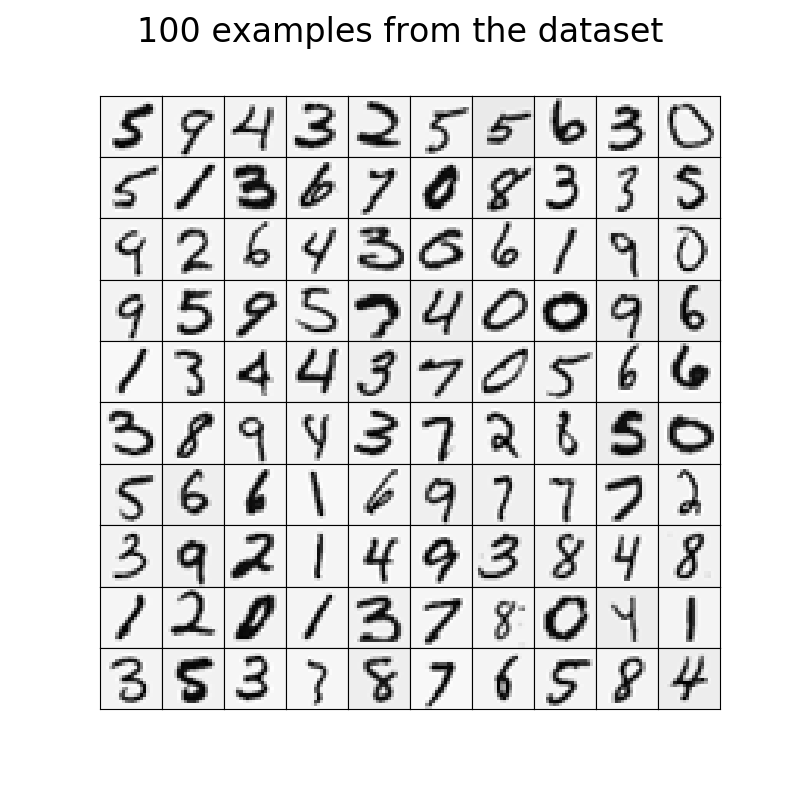#### Ex3.2 Neural Networks

Instruction:

In this part of the exercise, you will implement a neural network to recognize handwritten digits using the same training set as before. The neural network will be able to represent complex models that form non-linear hypotheses. Your goal is to implement the feedforward propagation algorithm to use our weights for prediction.

Our neural network is shown below. It has 3 layers – an input layer, a hidden layer and an output layer. Recall that our inputs are pixel values of digit images. Since the images are of size $20×20$, this gives us 400 input layer units (excluding the extra bias unit which always outputs +1). As before, the training data will be loaded into the variables $X$ and $y$.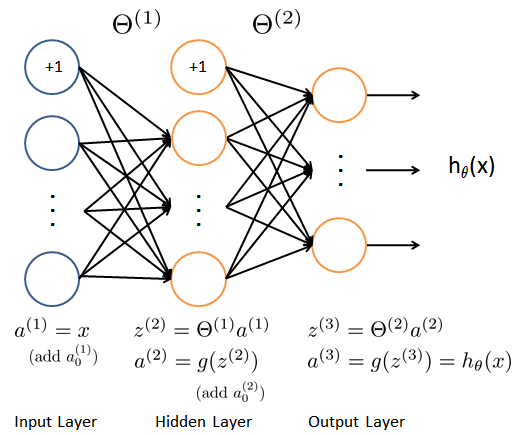Code:

1
2
3
4
5
6
7
8
9
10
11
12
13
14
15
16
17
18
19
20
21
22
23
24
25
26
27
28
29
30
31
32
33
34
35
36
37
38
39
40
41
42
43
44
45
46
47
48
49
50
51
52
53
54
55
56
57
58
59
60
61
62
63
64
65
66
67
68
69
import numpy as np
import matplotlib.pyplot as plt
import scipy.io as scio

''' Part 0: Functions and Parameters '''
X, y = data['X'], data['y']
m = len(y)
return X, y, m

# Randomly select several data points to display
def randomDisplay(data, num=100, cmap='binary', transpose=True):
sample = data[np.random.choice(len(data), num)]
size, size1 = int(np.sqrt(num)), int(np.sqrt(sample.shape))
fig0, ax0 = plt.subplots(nrows=size, ncols=size, sharex=True, sharey=True, figsize=(8, 8))
order = 'F' if transpose else 'C'
for i in range(size):
for j in range(size):
ax0[i, j].imshow(sample[size * i + j].reshape([size1, size1], order=order), cmap=cmap)
plt.xticks(np.array([]))
plt.yticks(np.array([]))
plt.suptitle(str(num)+' examples from the dataset', fontsize=24)

# Sigmoid function
def sigmoid(z):
return 1 / (1 + np.exp(-z))

# Predict the label of an input given a trained neural network
def predict(theta1, theta2, X):
a1 = np.c_[np.ones([X.shape, 1]), X]
z2 = np.dot(a1, theta1.T)
a2 = np.c_[np.ones([z2.shape, 1]), sigmoid(z2)]
z3 = np.dot(a2, theta2.T)
a3 = sigmoid(z3)
pred = np.argmax(a3, axis=1)
return pred + 1

# Setup the parameters
input_layer_size = 400  # 20x20 Input Images of Digits
hidden_layer_size = 25  # 25 hidden units
num_labels = 10         # 10 labels, form 1 to 10 (note that we have mapped '0' to label '10')

# Randomly select 100 data points to display
randomDisplay(X, 100, transpose=True)
plt.show()

theta1, theta2 = weights['Theta1'], weights['Theta2']

''' Part 3: Implement Predict '''
y_predict = predict(theta1, theta2, X)
print('\nTraining Set Accuracy: ', np.mean(y_predict == y.flatten()) * 100, '%')

# Randomly select examples
random_index = np.random.choice(m, size=10)
print('Indexes of examples :', random_index)
for i, idx in enumerate(random_index):
# print('Example[%d]\t is number %d,\t we predict it as %d' % (i + 1, y[idx], y_predict[idx    ]))
print('Example[{:0>2}] is number {:^2}, we predict it as {:^2}'.
format(str(i + 1), str(int(y[idx])), str(y_predict[idx])))


Output:

• Console: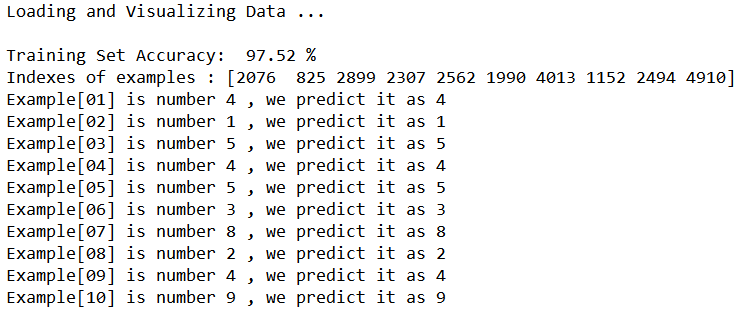Thistledown